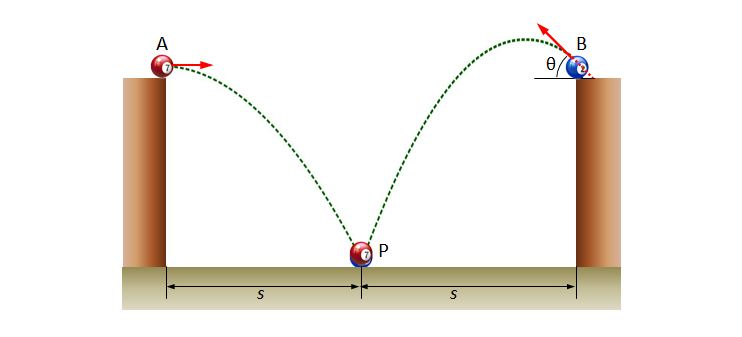# Projectile motion of ball 3A ball $A$ is thrown horizontally, and at the same time, another ball $B$ is thrown with an angle of $\theta$ to the horizontal line, as shown above. The horizontal throw lengths of the two balls are the same, which is $s$ in the picture, and the landing point of both balls is the point $P$ on the floor. Until the ball $A$ arrives at $P,$ which of the following statements is correct?

a) The two balls arrive at $P$ at the same time.

b) The accelerations of the two balls are the same.

c) The velocity of the ball $B$ relative to that of the ball $A$ is constant.

×